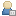###Show Posts

This section allows you to view all posts made by this member. Note that you can only see posts made in areas you currently have access to.

### Messages - Nvidia

Pages: 
1
##### Off-Topic (Read Only) / Need help for Programming a Timer 1 in P89V51RD2BN
« on: November 28, 2009, 02:21:37 am »
Aim : To generate a pulse of 4ms using a Timer1.(50% duty cycle) i.e. 4ms ON time and 4ms OFF time

Using Timer 1 the reload values calculated are  as follows

4ms*11.0592M = (FFFF- Reload value)*6
so there fore Reload value = E333.

This was the value I loaded into the program and when I saw output on P1.1 the ociloscope showed 8ms.

Now Problem : is (FFFF-Reloadvalue)*12 instead of 6.But data sheet shows osc/6.Here is my program : Please help me.

Code: [Select]
`sbit port = P1^1;   void main(void)   {    WDTC = 0x00; CMOD = 0x00; PCON = 0x00; P1 = 0xFF; TMOD = 0x10; TCON = 0x00; TL1 = 0x33; TH1 = 0xE3; TR1 = 1; // Start the Timer1 EA = 1; ET1 = 1; while(1) { TL1 = 0x33; TH1 = 0xEE; TR1 = 1; } } } void TIMER1_ISR(void) interrupt 3 { TF1 = 0; TR1 = 0; port = ~ port; }`

Please help me.Is there any mistake in my Code or the Data sheet is wrong.

Pages: